# Functions in Python

## Functions in Python with Examples

In this article, I am going to discuss Functions in Python with Examples. Please read our previous article where we discussed Debugging, Databases, and Project Skeletons in Python with Examples.

##### Functions in Python

A function in Python is a collection of related statements that performs a specific task. Functions aid in the division of our program into smaller, modular chunks. As our program grows in size, functions help to keep it organized and manageable. It also avoids repetition and makes the code reusable.

```def function_name(function):
"""docstring"""
statement(s)
```

The function definition shown above is made up of the following components:

1. The function header begins with the keyword def.
2. A function name that is unique to the function. The rules for naming functions in Python are the same as those for writing identifiers.
3. Parameters (arguments) are values that we pass to a function. They are entirely optional.
4. To indicate the end of the function header, use a colon (:).
5. Documentation string (docstring) is optional and used to describe what the function does.
6. The function body is made up of one or more valid Python statements. Statements must be indented at the same level (usually 4 spaces).
7. return statement, which is optional, is used to return a value from the function.
##### Example of a Function in Python
```def greeting(dotnet):
"""
This function greets to
the person passed in as
a parameter
"""
print("Hello, " + name + ". Good morning!")
```
##### In Python, how do you call a function?

Once a function has been defined, it can be called from another function, program, or even the Python prompt. To invoke a function, simply type the function name followed by the appropriate parameters.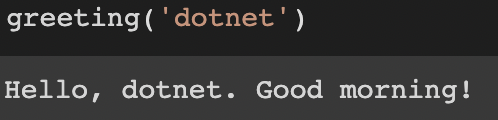Note: It is important to note that in Python, the function definition should always come before the function call. We’ll get an error if we don’t.

##### Docstrings in Python

The first string after the function header is referred to as the docstring, which is an abbreviation for documentation string. It is used to briefly describe what a function does.

Although it is not required, documentation is good programming practice. Always document your code, unless you remember what you had for dinner last week.

In the preceding example, a docstring appears immediately below the function header. We usually use triple quotes so that the docstring can span multiple lines. This string is available to us as the function’s __doc__ attribute.

##### The return Statement

The return statement is used to exit a function and return to the point where it was called from. This statement can include an expression, which is evaluated and the result is returned. If there is no expression in the statement or if the return statement is not present within a function, the function will return None.

##### The return Statement Example
```def abs_value(num):
"""This function returns the absolute
value of the entered number"""

if num >= 0:
return num
else:
return -num

print(abs_value(5))

print(abs_value(-3))
```
###### Output:##### Scope and lifetime of Variables in Python

A variable’s scope is the area of a program where the variable is recognized. Parameters and variables defined within a function are not visible from the outside. As a result, they have a limited scope. The duration of a variable’s existence in memory is defined as its lifetime. Variables within a function have a lifetime equal to the function’s execution time.

They are destroyed when we exit the function. As a result, a function does not recall the value of a variable from previous calls. Here’s an example of the scope of a variable within a function.

```def func():
x = 5
print("Value inside function:",x)

x = 14
func()
print("Value outside function:",x)
```
###### Output: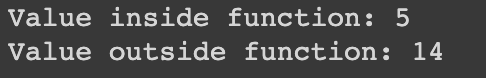In this case, we can see that the initial value of x is 14. Even though the function func() changed the value of x to 5, the value outside the function was unaffected. This is due to the fact that the variable x inside the function differs (is local to the function) from the one outside. Despite their similar names, they are two distinct variables with distinct scopes.

Variables outside of the function, on the other hand, are visible from within. They are global in scope. From within the function, we can read these values but not change (write) them. To change the value of variables outside the function, they must be declared as global variables with the global keyword.

Essentially, functions can be classified into two types:

1. Built-in functions are functions that are pre-installed in Python.
2. User-defined functions are those that are defined by the users themselves.
##### Function Parameters in Python

We learned about defining and calling functions in the user-defined function topic. Otherwise, the function call will fail with an error message. Here’s an illustration.

```def greeting(name, msg):
"""This function greets to
the person with the provided message"""
print("Hello", name + ', ' + msg)

greeting("Monica", "Good morning!")
```
###### Output: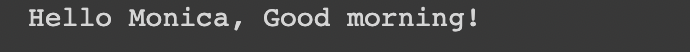The function greeting() has two parameters in this case. Because we called this function with two arguments, it runs smoothly and without error. The interpreter will display an error message if we call it with a different number of arguments. A call to this function with one and no arguments, as well as their respective error messages, is shown below.

greeting(“Monica”)

###### Output: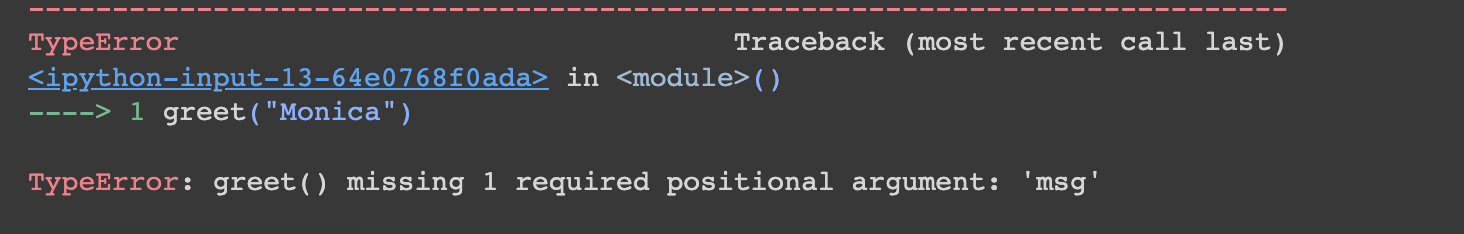##### Variable Function Arguments in Python

Functions had a fixed number of arguments up until now. There are other ways to define a function in Python that can take a variable number of arguments. This type is classified into three variants, which are described below.

##### Default Arguments in Python

In Python, function arguments can have default values. Using the assignment operator (=), we can provide a default value for an argument. Here’s an illustration.

```def greeting(name, msg="Good morning!"):
"""
This function greets to
the person with the
provided message.

If the message is not provided,
it defaults to "Good
morning!"
"""

print("Hello", name + ', ' + msg)

greet("Kate")
greet("Bruce", "How do you do?")
```
###### Output:The parameter name in this function does not have a default value and is required (mandatory) during a call. The parameter msg, on the other hand, has a default value of “Good morning!” As a result, it is optional during a call. If a value is supplied, it will replace the default value.

A default value can be assigned to any number of arguments in a function. However, once we have a default argument, all of the arguments to their right must have default values as well. This means that non-default arguments cannot be used after default arguments. For instance, suppose we had defined the function header above as:

def greeting(msg = “Good morning!”, name):

###### Output: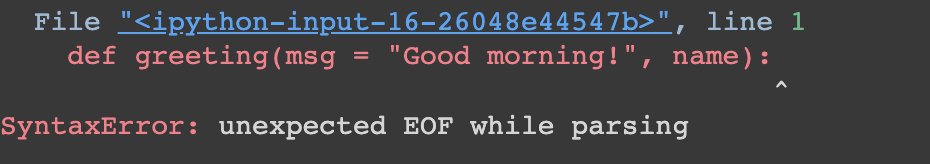##### Keyword Arguments in Python

When we call a function with some values, these values are assigned to the arguments in the order in which they appear. In the above function greet(), for example, when we call it greet(“Bruce”, “How do you do?”), the value “Bruce” is assigned to the argument name and “How do you do?” to msg.

Keyword arguments can be used to call functions in Python. When we call functions in this manner, we can change the order (position) of the arguments. All subsequent calls to the aforementioned function are valid and produce the same result.

```# 2 keyword arguments
greeting(name = "Bruce",msg = "How do you do?")

# 2 keyword arguments (out of order)
greeting(msg = "How do you do?",name = "Bruce")

#1 positional, 1 keyword argument
greeting("Bruce", msg = "How do you do?")
```
###### Output:##### Python Arbitrary Arguments

Sometimes we don’t know how many arguments will be passed into a function ahead of time. Python allows us to handle situations like this by using function calls with an arbitrary number of arguments. In the function definition, we use an asterisk (*) before the parameter name to denote this kind of argument. Here’s an illustration.

```def greeting(*names):
"""This function greets all
the person in the names tuple."""

# names is a tuple with arguments
for name in names:
print("Hello", name)

greeting("Monica", "Luke", "Steve", "John")
```
###### Output:##### Lambda Function in Python

Python Lambda Functions are anonymous functions, which means they don’t have a name. The def keyword is used to define a normal function in Python, as we already know. In Python, the lambda keyword is used to define an anonymous function.

Python Lambda Function Syntax: lambda arguments: expression

This function accepts any number of arguments but only evaluates and returns one expression. Lambda functions can be used wherever function objects are required.

It is important to remember that lambda functions are syntactically limited to a single expression. Aside from other types of expressions in functions, it has a variety of uses in specific fields of programming.

```string ='DotNet'
# lambda returns a function object
print(lambda string : string)
```
###### Output: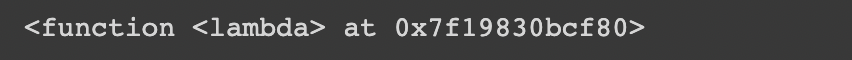In the preceding example, the lambda function is not called by the print function; instead, it simply returns the function object and the memory location where it is stored. So, in order for the print to print the string, we must first call the lambda so that the string can pass through the print.

##### Difference Between Lambda Functions and def defined function in Python

Let’s take a look at this example to see what the difference is between a normal def defined function and a lambda function. This program computes the cube of a given value:

```# showing difference between def() and lambda().
def cube(y):
return y*y*y
lambda_cube = lambda y: y*y*y
# using the normally
# defined function
print(cube(7))
# using the lambda function
print(lambda_cube(7))
```
###### Output:As we can see in the preceding example, the cube() and lambda cube() functions behave identically and as expected. Let’s take a closer look at the preceding example:

Without using Lambda, both of these functions return the cube of a given number. However, when using def, we needed to define a function with the name cube and pass it a value. We also needed to use the return keyword after execution to return the result from where the function was called.

##### Sorting Dictionaries in Python

Problem Statement – The following are the major tasks that must be completed.

1. Make a dictionary and arrange the keys alphabetically.
2. Display both the keys and the values alphabetically by key.
3. The same as in part (ii), but sorted alphabetically by value.

Approach – Load the Dictionary and run the following commands:

1. To begin, use key_value.iterkeys() function to sort the keys alphabetically.
2. Second, use the sorted (key-value) function to sort the keys alphabetically and print the value that corresponds to it.
3. Third, use key-value to sort the values alphabetically.

key = lambda (k, v): (v, k)) iteritems()

Let’s try completing the following tasks:

##### 1. Displaying the Keys in Alphabetical Order:
```# Function calling
def dictionairy():
# Declare hash function
key_value ={}
# Initializing value
key_value = 46
key_value = 22
key_value = 12
key_value = 32
key_value = 15
key_value = 213
print ("1:-\n")
print ("Keys are")
# iterkeys() returns an iterator over the
# dictionary’s keys.
for i in sorted (key_value.keys()) :
print(i, end = " ")
def main():
# function calling
dictionairy()

# Main function calling
if __name__=="__main__":
main()
```
###### Output: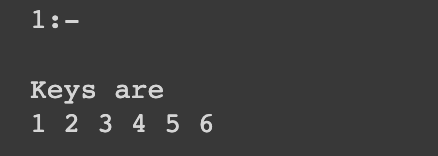##### 2. Using the Key, sort the Keys and Values in alphabetical order.
```# function calling
def dictionairy():
# Declaring the hash function
key_value ={}
# Initialize value
key_value = 46
key_value = 22
key_value = 12
key_value = 32
key_value = 15
key_value = 213
print ("Task 2:-\nKeys and Values sorted in",
"alphabetical order by the key  ")
# sorted(key_value) returns an iterator over the
# Dictionary’s value sorted in keys.
for i in sorted (key_value) :
print ((i, key_value[i]), end =" ")
def main():
# function calling
dictionairy()

# main function calling
if __name__=="__main__":
main()
```
###### Output: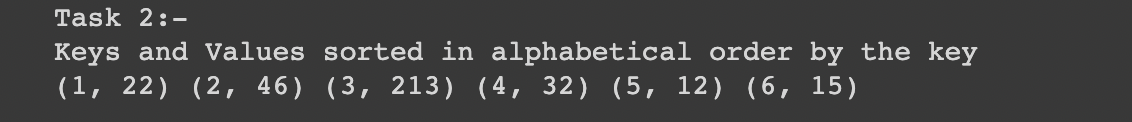##### 3. Sort the dictionary by key
```from collections import OrderedDict
dict = {'raj':'10','akash':'9','abhinav':'15','yashraj':'2','sumit':'32'}
dict1 = OrderedDict(sorted(dict.items()))
print(dict1)
```
###### Output: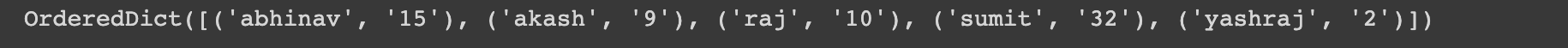##### Alternate Keys

When working with Python Dictionaries, we may encounter situations in which we need to assign a specific value to a specific key but, in the absence of that value, require a similar key’s value from a different dictionary. This problem has potential applications in the field of web development. Let’s go over some different approaches to completing this task.

##### Using Loop
```# initializing dictionary
test_dict = {'dotnet' : {'a' : 1, 'b' : 2, 'c' : 3}, 'good' : {'a' : 3, 'c' : 4}}
# printing original dictionary
print("The original dictionary is : " + str(test_dict))
# alternate key
alt_key = 'dotnet'
# Alternate Default Key Value
# Using loop
if 'b' in test_dict['good']:
res = test_dict['good']['b']
else :
res = test_dict[alt_key]['b']

# printing result
print("The required value : " + str(res))
```
###### Output:##### Using get()
```# initializing dictionary
test_dict = {'dotnet' : {'a' : 1, 'b' : 2, 'c' : 3}, 'good' : {'a' : 3, 'c' : 4}}
# printing original dictionary
print("The original dictionary is : " + str(test_dict))
# alternate key
alt_key = 'dotnet'
# Alternate Default Key Value
# Using get()
res = test_dict.get('good').get('b', test_dict.get(alt_key)['b'])

# printing result
print("The required value : " + str(res))
```
###### Output:##### Sorting Lists in Python:

By default, the sort() method ascends the list. You can also create a function that determines the sorting criteria.

```def myFunc(e):
return len(e)

cars = ['Ford', 'Mitsubishi', 'BMW', 'VW']

cars.sort(key=myFunc)
```
###### Output: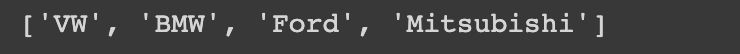##### Sorting a list of dictionaries in Python
```def myFunc(e):
return e['year']

cars = [
{'car': 'Ford', 'year': 2005},
{'car': 'Mitsubishi', 'year': 2000},
{'car': 'BMW', 'year': 2019},
{'car': 'VW', 'year': 2011}
]

cars.sort(key=myFunc)
```
###### Output: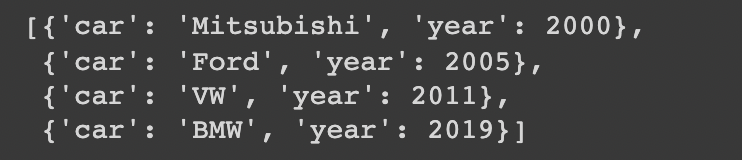##### Sorting Tuples in Python

Sorting tuples is actually less complex because they are immutable data structures, so there is less to consider when sorting them. You have no other option but to use the sorted() function to create a sorted copy of the input tuple.

```tuples = (8, 3, 4, 1, 5)
sorted_tuple = sorted(tuples)
sorted_tuple
```
###### Output: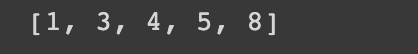In the next article, I am going to discuss Errors and Exception Handling in Python with Examples. Here, in this article, I try to explain Functions in Python with Examples. I hope you enjoy this Functions in Python article.